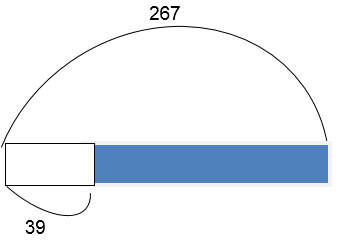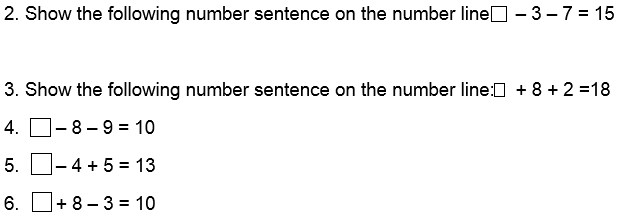# Lesson Notes By Weeks and Term - Primary 3

TERM: 1st Term

WEEK: 8

CLASS: Primary 3

AGE: 8 years

DURATION: 5 periods of 40 minutes each

DATE:

SUBJECT: Mathematics

SPECIFIC OBJECTIVES: At the end of the lesson, the pupils should be able to

1. To Solve addition and subtraction problems using bar diagrams

INSTRUCTIONAL TECHNIQUES: Explanation, question and answer, demonstration, practical, assessments

INSTRUCTIONAL MATERIALS: Base 10 kit, 100 number board, scrap paper, videos from source-

PERIOD 1: Word problems

 PRESENTATION TEACHER’S ACTIVITY PUPIL’S ACTIVITY STEP 1MENTAL MATHS The teacher begins the lesson with some mental calculationsCalculate1. 1 + 15 =2. 2 + 25 =3. 3 + 19 =4. 4 + 45 =5. 5 + 78 =6. 3 + 35 =7. 1 + 42 =8. 2 + 55 =9. 4 + 65 =10. 4 + 78 = Pupils respond and participate STEP 2CONCEPTDEVELOPMENT The teacher writes 348 + 26 = on the board. Learners are asked to solve this using simplified pictorials A learner is asked to show the solution to the question on the boardUsing simplified pictorialThe process is repeated for59 + 407 =941 – 22 =736 – 27 = Then the teacher writes the following word problem on the board“There are 129 sweets in the jar. Daniel puts in another 47 sweets. How many sweets are in the jar now? The teacher then draws the below diagramThe learners are then asked to discuss what the number sentence will be(129 + 47 = ____)Then the learners are allowed to solve the problem using their preferred method129 + 47 = 176.The teacher explains to the pupils that the word problem is an addition problem since the number of sweets are the ending is more than the number of sweets in the jar at the beginning  The teacher writes the below question on the boardThere are 267 children on the field. 39 go back to their classrooms. How many children are on the field now? The teacher draws the below diagram on the boardThe teacher asks the learners to think what the number sentence will be(267 – 39 = _____)The learners are then asked to work out the solution267 – 39 = 228 Pupils pay attention and participate STEP 3CLASS-WORK 1. Solve the word problem using bar diagramsa. The teacher has 342 pencils. The shop down the road gives her 69 more. How manypencils does she have now? b Theophilous picks up 543 shells. 29 were broken. How many unbroken shells does Theophilous have? Pupils attempt their class work STEP 4HOME-WORK 1. Solve the word problem using bar diagramsa. Segun picks up 264 pieces of litter. He then picks up another 17 pieces of litter. How many pieces of litter did Segun pick up altogether? Pupils attempt their class work STEP 5SUMMARY The teacher summarizes reminding the pupils of the steps in solving word problems using bar diagrams  She marks their class works, makes corrections where necessary and commends them positively

PERIOD 2: Revision of addition and subtraction

PRESENTATION

TEACHER’S ACTIVITY

PUPIL’S ACTIVITY

STEP 1

MENTAL MATHS

The teacher begins the lesson with some mental calculations

Calculate

1. 8 + 4 =

2. 3 + 9 =

3. 7 + 5 =

4. 4 + 8 =

5. 6 + 6 =

6. 14 – 5 =

7. 15 – 6 =

8. 16 – 7 =

9. 17 – 8 =

10. 18 – 9 =

Pupils respond and participate

STEP 2

CONCEPT

DEVELOPMENT

The teacher writes 351 – 35 = on the board. Learners are asked to solve this using the column method

A learner is asked to show the solution to the question on the board

Using simplified pictorial

 H T U 3 45 11 - 3 5 3 1 6

The same process is repeated for these

1. 673 – 47 =

2. 145 – 136 =

3. 107 - 28 =

Pupils pay attention and participate

STEP 3

CLASS-WORK

1. Use column method. Ensure to use the check to see whether or not the answer is correct

a. 142 – 74 =

b. 125 – 46 =

c. 687 – 59 =

d. 574 – 35 =

e. 105 – 58 =

Pupils attempt their class work

STEP 4

HOME-WORK

1. Use column method. Ensure to use the check to see whether or not the answer is correct

a. 126 – 89 =

b. 843 – 29 =

Pupils attempt their class work

STEP 5

SUMMARY

The teacher summarizes reminding the pupils of the steps in carrying out subtraction using the column method and how to check whether the solution is correct

She marks their class works, makes corrections where necessary and commends them positively

 PRESENTATION TEACHER’S ACTIVITY PUPIL’S ACTIVITY STEP 1ORALASSESSMENTS The teacher asks questions on mental maths with 2 and 3-digit numbers treated so far Pupils respond and participate STEP 2DISCUSSION The teacher discusses all the methods used by some learners in the oral assessments(some of the questions are solved on the board by the learners) and addresses any misconceptions that may have risen Pupils pay attention and participate STEP 3WRITTEN ASSESSMENTS 1 Solve the following using the column methoda. 58 + 59 =b. 96 + 27 =c. 156 - 69 =d. 882 - 65 =e. 106 – 9 = 2. Check to see if these subtractions are correct by adding thema. 487 – 28 = 437b. 262 – 48 = 214 3. Richard has N328. His mother gave him N48. How much does he have now? 4. There are 421 mails in my e-mail box. I deleted 64 mails. How many am I left with? Pupils attempt their class work STEP 4SUMMARY The teacher marks the written assessments, corrects were necessary and commends the pupils

PERIOD 4: Finding the missing number

 PRESENTATION TEACHER’S ACTIVITY PUPIL’S ACTIVITY STEP 1MENTAL MATHS The teacher begins the lesson with some mental calculationsCalculate1. 3 + 8 =2. 4 + 9 =3. 5 + 7 =4. 6 + 8 =5. 7 + 6 =6. 7 + 4 =7. 8 + 8 =8. 8 + 5 =9. 9 + 7 =10. 9 + 8 = Pupils respond and participate STEP 2CONCEPTDEVELOPMENT The teacher asks the learner to follow the below problem: I am thinking of a number. When I take 9 away from the number, my answer is 10. What number am I thinking of?__19__ - 9 = 10The teacher asks the learners to discuss their answers and how they got it The teacher writes another problem on the board:There were some learners in a classroom. 3 learners went out. Another 5 learners went out. Now there are 11 learners left in the classroom. How many learners were there at the beginning?The teacher writes the below sentencePupils pay attention and participate STEP 3CLASS-WORK Complete the following. You may want to draw a number line to help you9. What number do we add to 2 and 8 to get 15?10. What number do we add to 8 and 9 to get 20? Pupils attempt their class work STEP 4HOME-WORK Complete the followingThe pupils writes it in their homework book STEP 5SUMMARY The teacher reminding the pupils how addition can be used to solve subtraction problemsShe marks the class work, make corrections where necessary and commends the pupils positively

PERIOD 5: Weekly Test/consolidations

TEACHER’S ACTIVITY: The teacher revises all the concepts treated from period 1-4 and gives the pupils follow through exercises, quiz and tests . She marks the exercises, makes corrections and commends the pupils positively.

PUPIL’S ACTIVITY: The pupils work on the worksheets and exercises given by the teacher individually

ASSESSMENT

1. I am thinking of a number. When I take 7 away from the number my answer is 11.

What number am I thinking of? ___